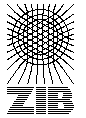# Testset Relay

The instances are derived from 3D placement of unmanned aerial vehicles used for multi-target surveillance, as tested by Burdakov et al. (2014) liu.diva-portal.org. While the number of terminals is relatively small, the amount of nodes and especially arcs is tremendous.

The original data sets are from the liu.diva-portal.org.

Name   |V|    |E|    |T|    DC    Opt

The column DC classifies the difficulty of the instance.

L
Solvable by usage of local preprocessing. Typical examples are the SD-Test, BD-n Tests and FST computations. Neither a global upper nor lower bound needs to be computed.
P
Solvable by polynomial time algorithms, like dual ascent in combination with primal heuristic, a integral LP formulation or advanced preprocessing like reduced cost criteria or the RCR-Test.
NP
No polynomial time algorithm is known. Use of an exponential time enumeration sceme like Branch-and-Bound is neccessary.

The letter after class gives an impression how long it takes to solve the problem using state-of-the-art soft- and hardware. secounds means less than a minute (this includes instances which can be solved in fractions of a second). minutes means less than an hour. hours is less than a day and days is less than a week. weeks mean it takes really a long time to solve this instance. ? means the instance is not solved or the time is not known.

If the number in the Opt column is written in italics the optimum is not known. The number given is the best know upper bound.

Last Update : 2015/02/11 11:57:20 \$ by Thorsten Koch